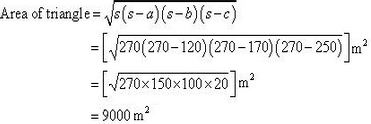# RD SHARMA Solutions for Class 9 Maths Chapter 17 - Heron's Formula

Page / Exercise

## Chapter 17 - Heron's Formula Ex. 17.1

Solution 1Solution 2Solution 3Solution 4Solution 5
The sides of the triangular field are in the ratio 25:17:12.
Let the sides of triangle be 25x, 17x, and 12x.
Perimeter of this triangle = 540 m
25x + 17x + 12x = 540 m
54x = 540 m
x = 10 m
Sides of triangle will be 250 m, 170 m, and 120 m.

Semi-perimeter (s) =By Heron's formula:So, area of the triangle is 9000 m2.
Solution 6Solution 7Solution 8Solution 9Solution 10Solution 11## Chapter 17 - Heron's Formula Ex. 17.2

Solution 1ForABC
AC2 = AB2 + BC2
(5)2 = (3)2 + (4)2
So,ABC is a right angle triangle, right angled at point B.
Area ofABCForADC
Perimeter = 2s = AC + CD + DA = (5 + 4 + 5) cm = 14 cm
s = 7 cm
By Heron's formula
Area of triangleArea of ABCD = Area ofABC + Area ofACD
= (6 + 9.166) cm2 = 15.166 cm2 = 15.2 cm2 (approximately)

Solution 2Solution 3Solution 4
Let us join BD.
InBCD applying Pythagoras theorem
BD2 = BC2 + CD2
= (12)2 + (5)2
= 144 + 25
BD2 = 169
BD = 13 mArea ofBCDForABDBy Heron's formulaArea of triangleArea of park = Area ofABD + Area ofBCD
= 35.496 + 30 m2
= 65.496 m2
= 65. 5 m2 (approximately)

Solution 5Solution 6Solution 7Solution 8Solution 09Solution 10Solution 11Solution 12Solution 13Solution 14## Chapter 17 - Heron's Formula 17.24

Solution 1Solution 2Solution 3Solution 4Solution 5## Chapter 17 - Heron's Formula 17.25

Solution 6Solution 7Solution 21Solution 22Solution 23Solution 25Solution 8Solution 9Solution 24Solution 26### STUDY RESOURCES

REGISTERED OFFICE : First Floor, Empire Complex, 414 Senapati Bapat Marg, Lower Parel, Mumbai - 400013, Maharashtra India.